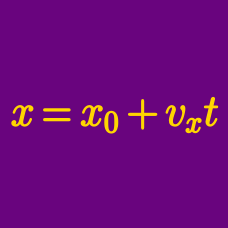Classical Mechanics

# Position vs. time

Ball $A$ is projected vertically upwards with a certain initial speed. Ball $B$ that is equal to $A$ in mass, is projected at the same initial speed in a direction that makes a $60^{\circ}$ angle with the vertical axis. What is the ratio of their potential energies at their respective highest points?

Ignore any air resistance.

From the top of a tower of height $105 \text{ m},$ a ball is projected upwards with a speed of $40 \text{ m/s}$ at $30^\circ$ angle with the horizon. If air resistance is negligible, what is the approximate horizontal displacement of the ball from the moment the ball is projected to the point the ball hits the ground?

Assume that $\sqrt{3}$ is approximately $1.7,$ and the gravitational acceleration is $g= 10 \text{ m/s}^2.$

From the top of a tower of height $60 \text{ m},$ a ball is projected upwards with a speed of $40 \text{ m/s}$ in a direction that makes a $30^\circ$ angle with the horizon. How many seconds will it take for the ball to hit the ground?

The gravitational acceleration is $g= 10 \text{ m/s}^2.$

A $700 \text{ g}$ ball is thrown in a direction that makes a $30^\circ$ angle with the horizon. If the ball's peak height is $45 \text{ m},$ how fast was the ball thrown?

The gravitational acceleration is $g= 10 \text{ m/s}^2.$

A body is projected horizontally from a point above the ground. The motion of the body is described by the following equations: $\begin{array}{c}&x= 6t &y = 5t^2, \end{array}$ where $x$ and $y$ are the horizontal and vertical displacements (in $\text{m}$), respectively, at time $t.$ What shape is the trajectory of the body?

×